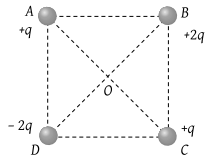The law, governing the force between electric charges is known as

(1) Ampere's law

(2) Ohm's law

(4) Coulomb's law

Concept Questions :-

Coulomb's law
High Yielding Test Series + Question Bank - NEET 2020

Difficulty Level:

Fg and Fe represents gravitational and electrostatic force respectively between electrons situated at a distance 10 cm. The ratio of Fg/ Fe is of the order of

(1) 1042

(2) 10

(3) 1

(4) 10–43

Concept Questions :-

Coulomb's law
High Yielding Test Series + Question Bank - NEET 2020

Difficulty Level:

A soap bubble is given a negative charge, then its radius

(1) Decreases

(2) Increases

(3) Remains unchanged

(4) Nothing can be predicted as information is insufficient

Concept Questions :-

Coulomb's law
High Yielding Test Series + Question Bank - NEET 2020

Difficulty Level:

Four charges are arranged at the corners of a square ABCD, as shown in the adjoining figure. The force on the charge kept at the centre O is(1) Zero

(2) Along the diagonal AC

(3) Along the diagonal BD

(4) Perpendicular to side AB

Concept Questions :-

Coulomb's law
High Yielding Test Series + Question Bank - NEET 2020

Difficulty Level:

In the absence of other conductors, the surface charge density

(1) Is proportional to the charge on the conductor and its surface area

(2) Inversely proportional to the charge and directly proportional to the surface area

(3) Directly proportional to the charge and inversely proportional to the surface area

(4) Inversely proportional to the charge and the surface area

Concept Questions :-

Electric field
High Yielding Test Series + Question Bank - NEET 2020

Difficulty Level:

Out of gravitational, electromagnetic, Vander Waals, electrostatic and nuclear forces; which two are able to provide an attractive force between two neutrons

(1) Electrostatic and gravitational

(2) Electrostatic and nuclear

(3) Gravitational and nuclear

(4) Some other forces like Vander Waals

High Yielding Test Series + Question Bank - NEET 2020

Difficulty Level:

A total charge Q is broken in two parts Q1 and Q2 and they are placed at a distance R from each other. The maximum force of repulsion between them will occur, when

(1) ${Q}_{2}=\frac{Q}{R},\text{\hspace{0.17em}}{Q}_{1}=Q-\frac{Q}{R}$

(2) ${Q}_{2}=\frac{Q}{4},\text{\hspace{0.17em}}{Q}_{1}=Q-\frac{2Q}{3}$

(3) ${Q}_{2}=\frac{Q}{4},\text{\hspace{0.17em}}{Q}_{1}=\frac{3Q}{4}$

(4) ${Q}_{1}=\frac{Q}{2},\text{\hspace{0.17em}}{Q}_{2}=\frac{Q}{2}$

Concept Questions :-

Coulomb's law
High Yielding Test Series + Question Bank - NEET 2020

Difficulty Level:

Three charges 4q, Q, and q are in a straight line in the position of 0, l/2, and l respectively. The resultant force on q will be zero if Q =

(1) – q

(2) –2q

(3) $-\frac{q}{2}$

(4) 4q

Concept Questions :-

Coulomb's law
High Yielding Test Series + Question Bank - NEET 2020

Difficulty Level:

Two small spheres each having the charge +Q are suspended by insulating threads of length L from a hook. This arrangement is taken in space where there is no gravitational effect, then the angle between the two suspensions and the tension in each will be

(1) ${180}^{o},\text{\hspace{0.17em}}\frac{1}{4\pi {\epsilon }_{0}}\frac{{Q}^{2}}{{\left(2L\right)}^{2}}$

(2) ${90}^{o},\text{\hspace{0.17em}}\frac{1}{4\pi {\epsilon }_{0}}\frac{{Q}^{2}}{{L}^{2}}$

(3) ${180}^{o},\text{\hspace{0.17em}}\frac{1}{4\pi {\epsilon }_{0}}\frac{{Q}^{2}}{2{L}^{2}}$

(4) ${180}^{o},\text{\hspace{0.17em}}\frac{1}{4\pi {\epsilon }_{0}}\frac{{Q}^{2}}{{L}^{2}}$

Concept Questions :-

Coulomb's law
High Yielding Test Series + Question Bank - NEET 2020

Difficulty Level:

Two charges each of 1 coulomb are at a distance 1 km apart, the force between them is

(1) 9 × 103 Newton

(2) 9 × 10–3 Newton

(3) 1.1 × 10–4 Newton

(4) 104 Newton

Concept Questions :-

Coulomb's law